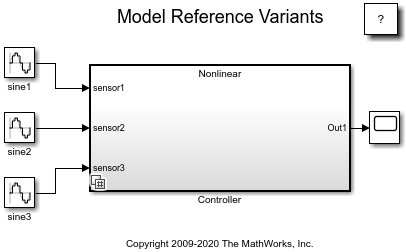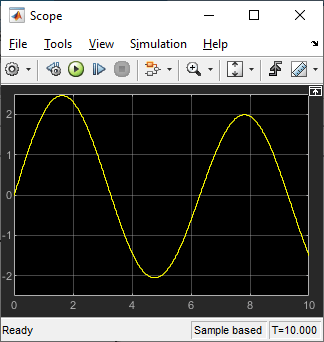Main Content

# Model Reference Variants

This example shows how to use model reference variants. A Model block is used to reference one Simulink® model from another Simulink model. A Variant Subsystem block can contain Model blocks as variants. A variant describes one of N possible modes in which a Variant Subsystem block can operate. Each variant references a specific model with its associated model-specific arguments. For a given Variant Subsystem block, only one variant is active during simulation. You can switch the active variant by changing the values of variables in the base workspace or by manually overriding variant selection using the Variant Subsystem block dialog box.

### Switching Active Variants

The `sldemo_mdlref_variants` model contains a Variant Subsystem block named Controller that references two models.

`open_system('sldemo_mdlref_variants')`To see the variant choices, right-click the Variant Subsystem block named Controller and select Block Parameters (Subsystem). The active variant is determined by the value of the `CTRL` variable in the MATLAB® base workspace. Defining `CTRL=1` activates the Model block named Linear, and defining `CTRL=2` activates the Model block named Nonlinear.

Opening the `sldemo_mdlref_variants` model runs the PreLoadFcn model callback, which sets `CTRL` to `2`.To simulate using the linear controller, define `CTRL=1` in the base workspace, then simulate the model.

```CTRL=1; sim('sldemo_mdlref_variants');```To simulate using the nonlinear controller, define `CTRL=2` in the base workspace, then simulate the model.

```CTRL=2; sim('sldemo_mdlref_variants');```### Enumerations and Reuse

The `sldemo_mdlref_variants_enum` model demonstrates `Simulink.Variant` object capabilities:

• Enumerations: MATLAB enumeration classes can be used to improve the readability of the conditions of the `Variant` object.

• Reuse: `Variant` objects can be reused in different Variant Subsystem blocks.

The Variant Subsystem blocks in this model require the following variables be defined in the MATLAB base workspace:

• `VE_LINEAR_CONTROLLER = Simulink.Variant('E_CTRL==sldemo_mrv_CONTROLLER_TYPE.LINEAR')`

• `VE_NONLINEAR_CONTROLLER = Simulink.Variant('E_CTRL==sldemo_mrv_CONTROLLER_TYPE.NONLINEAR')`

• `E_CTRL = sldemo_mrv_CONTROLLER_TYPE.LINEAR`

• `VE_PROTOTYPE = Simulink.Variant('E_CURRENT_BUILD==sldemo_mrv_BUILD_TYPE.PROTOTYPE')`

• `VE_PRODUCTION = Simulink.Variant('E_CURRENT_BUILD==sldemo_mrv_BUILD_TYPE.PRODUCTION')`

• `E_CURRENT_BUILD = sldemo_mrv_BUILD_TYPE.PRODUCTION`

Each variant is associated with a `Simulink.Variant` object that uses either the `sldemo_mrv_BUILD_TYPE.m` or `sldemo_mrv_CONTROLLER_TYPE.m` enumeration class.

The `Variant` objects have a `Condition` property that is an expression. The expression evaluates to a Boolean and determines which variant is active. The expression can consist of scalar variables, enumerations, equality, inequality, &&, ||, and ~. Parenthesis () can be used for precedence grouping. Variables may be standard MATLAB variables or `Simulink.Parameter` objects.

Opening the model `sldemo_mdlref_variants_enum` runs the PreLoadFcn model callback. This callback is set up to populate the base workspace with the variables required by the Variant Subsystem blocks.

`open_system('sldemo_mdlref_variants_enum')`The `VE_PROTOTYPE` and `VE_PRODUCTION` `Variant` objects are reused across the Variant Subsystem blocks named Filter1, Filter2, and Filter3.

The Block Parameters dialog box of the Variant Subsystem blocks show the Condition property of the related `Variant` objects.

## Support

#### Model-Based Design for Embedded Control Systems

Download white paper